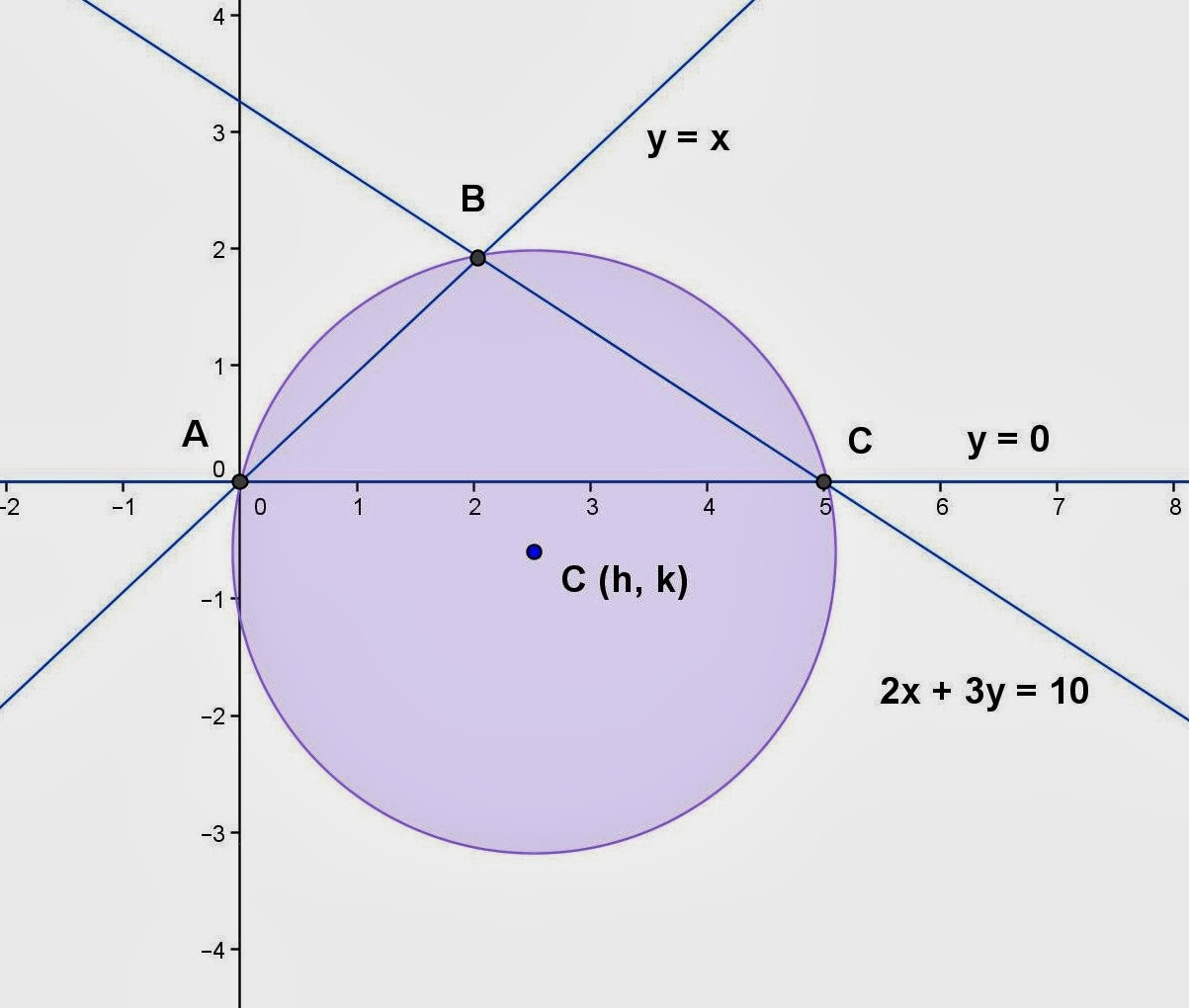## Sunday, February 2, 2014

### Finding Equation - Circle, 11

Category: Analytic Geometry, Plane Geometry, Algebra

"Published in Vacaville, California, USA"

Find the equation of a circle if it is inscribed in the triangle determined by the lines y = 0, 3x - 4y + 30 = 0, and 4x + 3y = 60.

Solution:

To illustrate the problem, it is better to draw the figure as followsA circle that is inscribed in a triangle from the three lines. (Photo by Math Principles in Everyday Life)

The first thing that we need to do is to get the equations of the angle bisectors of the given lines in order to get the coordinates of the center of a circle as well as its radius.A circle that is inscribed in a triangle from the three lines. (Photo by Math Principles in Everyday Life)

By using the distance of a point to the line formula, the angle bisector of lines y = 0 and 3x - 4y + 30 = 0 will be equal to

By using the distance of a point to the line formula, the angle bisector of lines y = 0 and 4x + 3y = 60 will be equal to

Hence, the angle bisectors are

Two angle bisectors are enough to use in order to get their intersection which is the center of a circle. Subtract the second equation from the first equation, we have

Substitute the value of y to either of the two equations above, we have

The coordinates of the center of a circle is C (5, 5). Since the center of a circle is located above the side of a triangle which is also the y-axis, then the radius of a circle is equal to 5.

Therefore, the equation of a circle is

## Saturday, February 1, 2014

### Finding Equation - Circle, 10

Category: Analytic Geometry, Plane Geometry, Algebra

"Published in Vacaville, California, USA"

Find the equation of a circle that is circumscribes a triangle determined by the lines y = 0, y = x, and 2x + 3y = 10.

Solution:

To illustrate the problem, it is better to draw the figure as followsA circle that is circumscribes a triangle from the intersection of three lines. (Photo by Math Principles in Everyday Life)

The first thing that we need to do is to get the intersections of three lines that passes the curve of a circle.

To get the coordinates of A, use equations y = 0 and y = x as follows:

Since the intersection of y = 0 and y = x is (0, 0) as shown from the figure, then the first point of intersection is A (0, 0).

To get the coordinates of B, use equations y = x and 2x + 3y = 10 as follows:

but

then the above equation becomes,

If x = 2, then y = 2. Hence, the second point of intersection is B (2, 2).

To get the coordinates of C, use equations y = 0 and 2x + 3y = 10 as follows:

but

then the above equation becomes,

Hence, the third point of intersection is C (5, 0).

The general equation of a circle is

Since the three points of a circle are given, then we can solve for the values of D, E, and F.

If you will use A (0, 0), then the above equation becomes

If you will use C (5, 0), then the above equation becomes

but F = 0, then the above equation becomes

If you will use B (2, 2), then the above equation becomes

Substitute D = - 5 and F = 0, we have

Therefore, the equation of a circle is